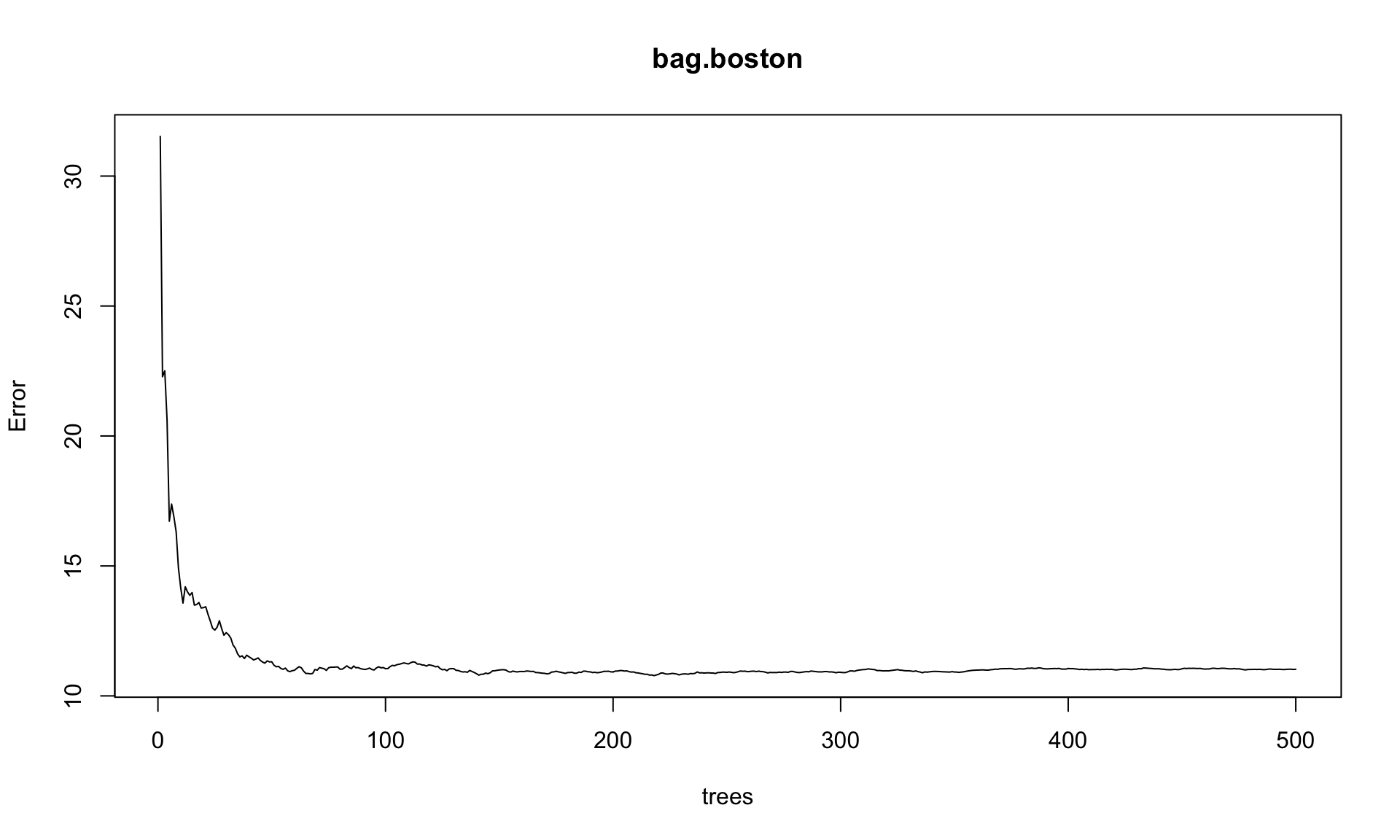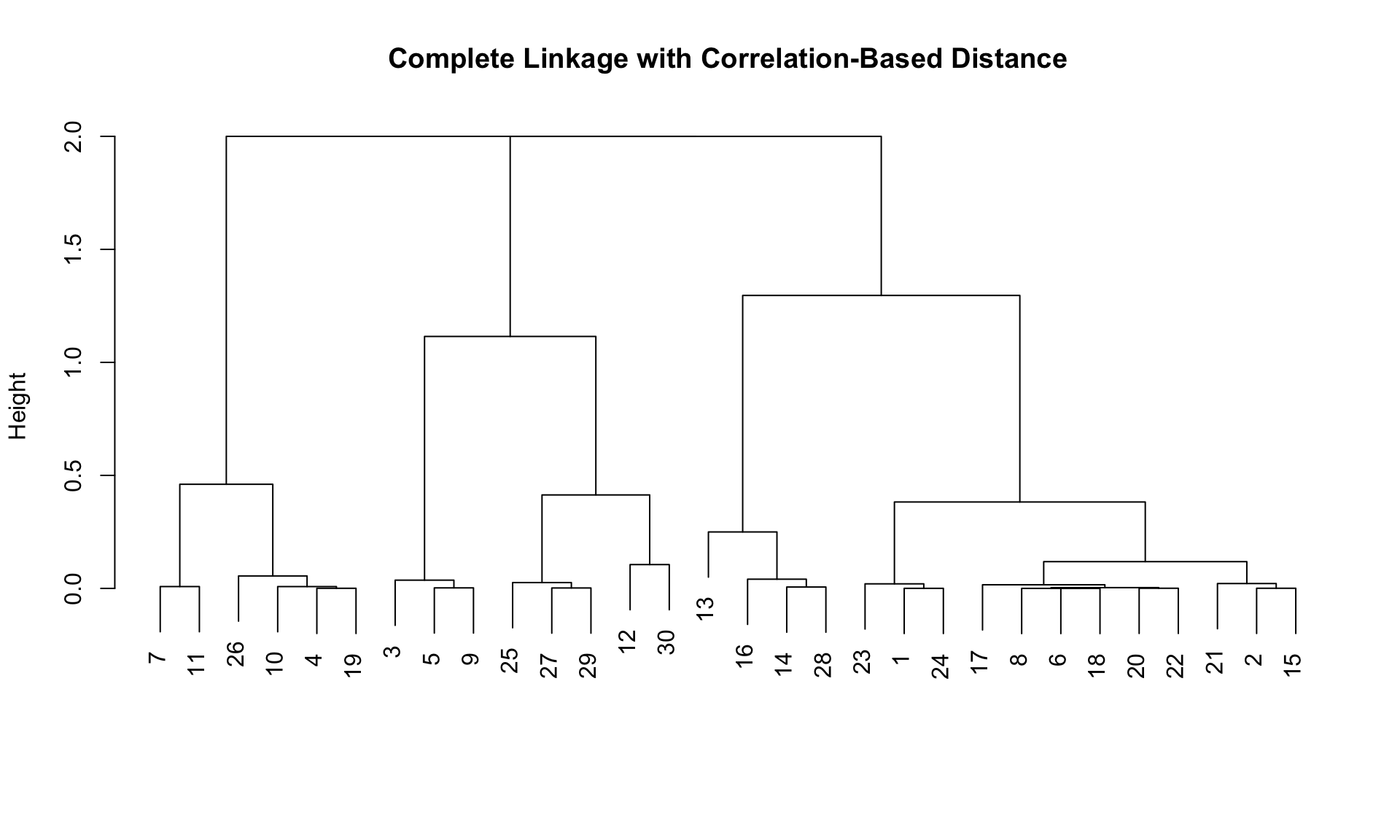library(ggplot2) # graphics library
library(ISLR)    # contains code and data from the textbook
library(knitr)   # contains knitting control
library(tree)    # For the tree-fitting 'tree' function
library(MASS)    # For Boston data
library(randomForest) # For random forests and bagging
## randomForest 4.6-12
## Type rfNews() to see new features/changes/bug fixes.
##
## Attaching package: 'randomForest'
## The following object is masked from 'package:ggplot2':
##
##     margin
library(gbm)  # For boosting
## Loading required package: survival
## Loading required package: lattice
## Loading required package: splines
## Loading required package: parallel
## Loaded gbm 2.1.1
options(scipen = 4)  # Suppresses scientific notation

### 1. Changing the author field and file name.

##### (b) Rename this file to “lab05_YourHameHere.Rmd”, where YourNameHere is changed to your own name.

The next portion of the lab gets you to carry out the Lab exercises in §8.3.2, 8.3.3, 10.5.1, 10.5.2 of ISL. You will want to have the textbook Lab open in front you as you go through these exercises. The ISLR Lab provides much more context and explanation for what you’re doing.

### 2. Random forests and Boosting

You will need the Carseats data set from the ISLR library in order to complete this exercise.

Please run all of the code indicated in §8.3.3-8.3.4 of ISLR, even if I don’t explicitly ask you to do so in this document.

##### (a) Run the View() command on the Carseats data to see what the data set looks like.
#View(Carseats)
##### The following code construst the High variable for the purpose of classification. Our goal will be to classify whether Carseat sales in a store are high or not.
High <- with(Carseats, ifelse(Sales <= 8, "No", "Yes"))
Carseats <- data.frame(Carseats, High)

# Split into training and testing
set.seed(2)
train <- sample(1:nrow(Carseats), 200)
Carseats.test <- Carseats[-train,]
High.test <- High[-train]
##### By setting mtry = p in the randomForest command, we can use the randomForest function to fit a bagged tree model. Here’s what we get.
# Define training set
set.seed(1)
train <- sample(1:nrow(Boston), nrow(Boston)/2)
boston.test <- Boston\$medv[-train]

set.seed(1)
bag.boston <- randomForest(medv ~ .,data=Boston, subset=train, mtry=13, importance=TRUE)
bag.boston
##
## Call:
##  randomForest(formula = medv ~ ., data = Boston, mtry = 13, importance = TRUE,      subset = train)
##                Type of random forest: regression
##                      Number of trees: 500
## No. of variables tried at each split: 13
##
##           Mean of squared residuals: 11.02509
##                     % Var explained: 86.65
##### (a) As always, we can use the plot command on a fitted model object to get some kind of plot of the model. This plot shows the Out-of-bag MSE on the y-axis. What is the plot showing on the x-axis?
plot(bag.boston)##### (b) Now let’s try fitting an actual random forest. The default setting is to take mtry = p/3 for regression and mtry = sqrt(p) for classification. Try fitting a random forest to the training data using mtry = 6. Call your fit rf.boston. Calculate its test set MSE.
# Edit me
##### (c) Run importance and varImpPlot on your random forest fit. How many plots are produced by the varImpPlot command? What are these plots showing?
# Edit me
##### (d) Use the partialPlot command to get partial dependence plots for the 2 most important variables according to the %IncMSE importance. Describe what you see.
# Edit me
##### (e) Using the gbm function, apply boosting with 5000 trees, allowing for trees up to depth 4. Fit to just the training data. You’ll need to specify distribution = "gaussian". Call your object boost.boston, and run the summary() command on it.
set.seed(1)
# Edit me

Note: The summary() command will produce a plot of each variable’s relative influence. This should be interpreted just like the 2nd importance measure for random forests: It’s the decrease in error from splitting on the variable, averaged over all the trees.

##### (f) Use the plot command to get partial dependence plots for rm and lstat. How do these compare to the partial dependence plots from the random forest fit?
par(mfrow=c(1,2))
# Edit me
##### (g) Use your boosted model to predict outcomes for the testing data. Calculate the MSE. How does it compare to random forests?
# Edit me
##### (h) Let’s try increasing the learning rate. The default value is shrinkage = 0.001. What happens if we take shrinkage = 0.2? Fit this model, keeping all other settings the same. Calculate the test MSE. Compare to the test MSE from the earlier model.
# Edit me
##### (i) Let’s try speeding up the learning even more. Take shrinkage = 0.7. Calculate test MSE, and compare to the previous two versions.
# Edit me
##### (j) gbm has built-in Cross-validation functionality. Set the argument cv.folds = 10 to perform cross-validation. Call your object boost.boston.cv. Use the default shrinkage settings.
# Edit me
##### (k) boost.boston.cv now has an attribute called cv.error. This attribute gives the 10-fold CV error for each tree size. Plot the CV error against tree size. What number of trees gives the lowest CV error? What is the minimum CV error achieved by boosting?
# Edit me
##### (l) Repeat parts (j) and (k), this time with shrinkage = 0.2. Comment on your findings.
# Edit me

### 3. K-means clustering

Here’s the small data set that’s generated in the book. This is a very stylized example, but it’s good enough for the purpose of introducing you to the basic clustering functions in R.

set.seed(2)
# generate data
x <- matrix(rnorm(50*2), ncol=2)
# shift the first 25 points to have mean (3, -4) instead of (0, 0)
x[1:25, 1] <- x[1:25, 1] + 3
x[1:25, 2] <- x[1:25, 2] - 4
##### (a) Plot the data in two dimensions. Colour it based on the true class label. The first 25 observations are from one class, and the next 25 points are from another class.
# Edit me
##### (b) Use the kmeans command to cluster the data into 2 classes. Specify nstart = 10. What does the nstart parameter mean?
# Edit me
##### (c) Compare the clustering you get to the true class labels. How well did we do?
# Edit me
##### (d) Now try splitting the data into 3 clusters. Plot your results. Does this look like a reasonable partition?
# Edit me
##### (e) Now try running K-means with 3 clusters and nstart = 1. Try it a bunch of times. Do you always get the same answer?
# Edit me

### 4. Hierarchical clustering

We’re going to continue using the same simple dataset as in Problem 3. But this time we’re going to learn about the hclust command for hierarchical clustering.

##### (a) Use the hclust command with different choices of method to perform complete, average, and single linkage clustering of the data. Note that the hclust function requires a distance matrix as the first argument. You will want to pass in dist(x), not x, to the hclust command.
# Edit me
##### (b) Plot the dendrograms for all three clusterings.
par(mfrow=c(1,3))
# Edit me
##### (c) Cut all of the dendrograms taking k = 2. You will notice from the documentation ?cutree that you can cut a dendrogram either by specifying the number of clusters you want, or the height you wish to cut at. Comment on the quality of the clusters.
# Edit me
##### (d) Construct plots for all 3 clusterings. Colour the points according to which cluster they wound up with.
# Edit me
##### (d) Does taking k = 4 with single linkage do any better? Plot your clustering.
# Edit me
par(mfrow = c(1,1))
plot(hclust(dd, method="complete"), main="Complete Linkage with Correlation-Based Distance", xlab="", sub="")